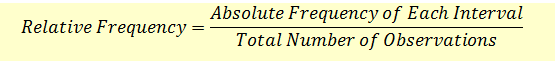# Relative Frequencies and Cumulative Relative Frequencies

The data in a frequency distribution can also be presented using relative frequencies.Once we have relative frequencies, we can calculate cumulative relative frequencies where as we move from first frequency interval to the last, we keep adding the relative frequencies finally reaching 100%. Cumulative relative frequencies are useful in measuring what fraction of total observations are less than the upper limit of a frequency interval.

We will extend our example to show the relative frequencies and cumulative relative frequencies.

 Interval Absolute Frequency Relative Frequencies Cumulative Relative Frequencies 0 <= r < 2 3 3/20 = 15% 15% 2 <= r < 4 5 5/20 = 25% 40% 4 <= r < 6 6 6/20 = 30% 70% 6 <= r < 8 2 2/20 = 10% 80% 8 <= r < 10 4 4/20 = 20% 100% 20 100%

The cumulative relative frequency is equal to the some of the relative frequencies of all the previous intervals including the current interval. For example, the cumulative absolute frequency for the interval 4 <= r < 6 is 15% + 25% + 30% = 70%.